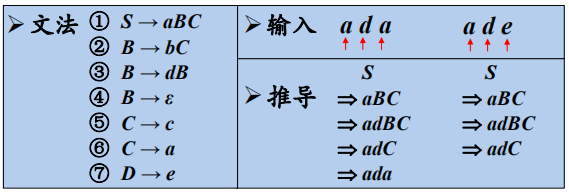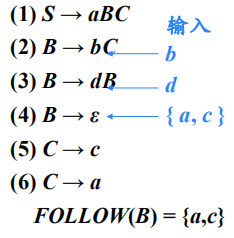### S_文法

S_文法（简单的确定性文法，Korenjak & Hopcroft，1966）

• 每个产生式的右部都以终结符开始

• 同一非终结符的各个候选式的首终结符都不同

S_文法不含ε产生式### 非终结符的后继符号集

FOLLOW(A)={a| S => αAaβ, a∈VT，α,β∈(VT∪VN)}### 产生式的可选集

• SELECT( A→aβ ) = { a }
• SELECT( A→ε )=FOLLOW( A )

q_文法：

• 每个产生式的右部或为ε ，或以终结符开始
• 具有相同左部的产生式有不相交的可选集

q_文法不含右部以非终结符打头的产生式

### 串首终结符集

• 对于 ∀α∈(VT∪V N)+, FIRST(α)={ a | α => aβ，a∈ VT，β∈(V T∪ V N)}；

• 如果 α =>* ε，那么 ε∈FIRST(α)

• 如果 ε∉FIRST(α), 那么SELECT(A→α)= FIRST(α)

• 如果 ε∈FIRST(α), 那么SELECT(A→α)=( FIRST(α)-{ε} )∪FOLLOW(A)

### LL(1)文法

• 如果α 和β均不能推导出ε ，则FIRST (α)∩FIRST (β) =Φ
• α 和β至多有一个能推导出ε
• 如果 β => ε，则FIRST (α)∩FOLLOW(A) =Φ；
如果 α =>
ε，则FIRST (β)∩FOLLOW(A) =Φ；

“1”表示在每一步中只需要向前看一个输入符号来决定语法分析动作